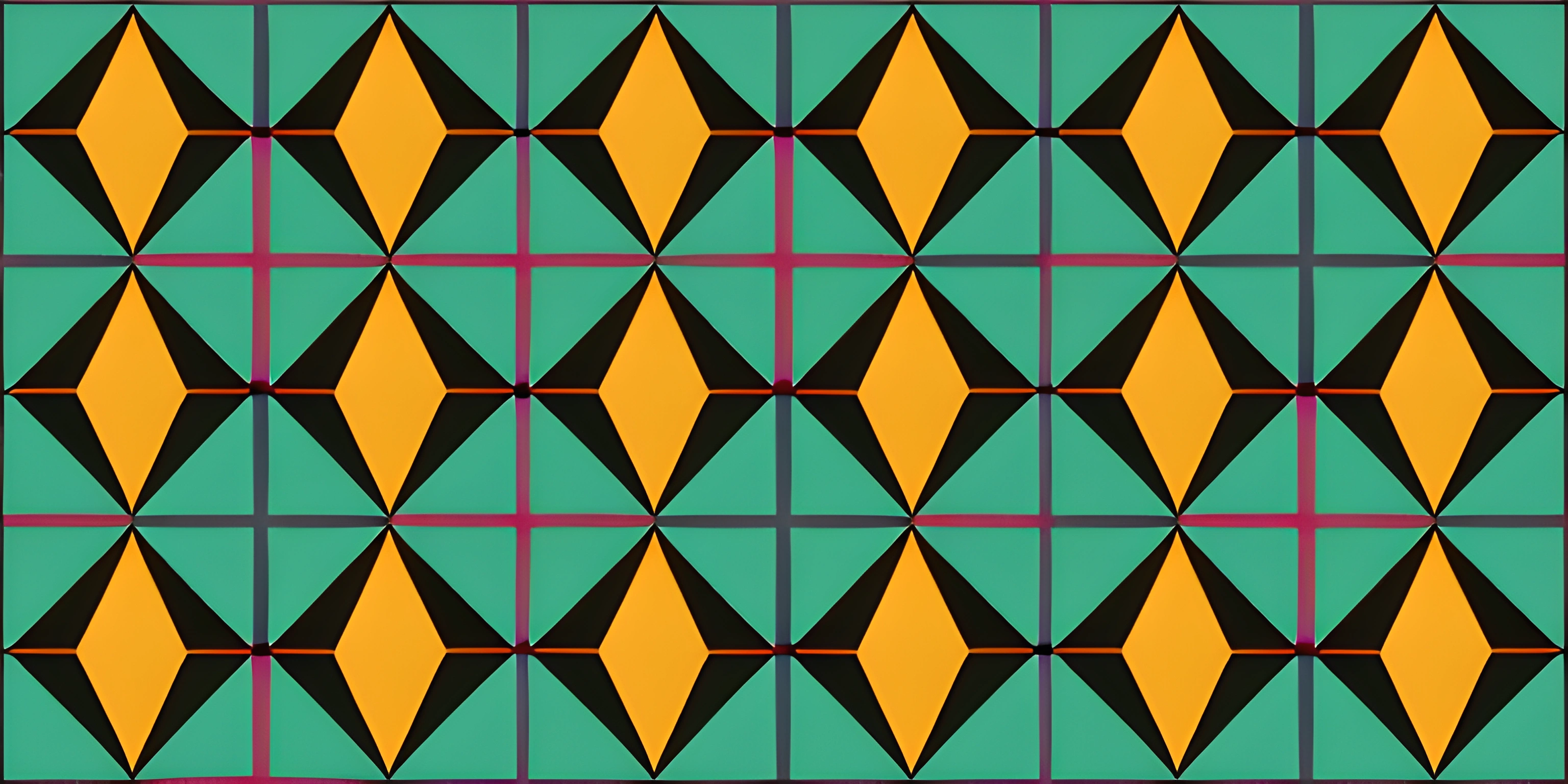# Vectors in p5.jsNote: this page has been created with the use of AI. Please take caution, and note that the content of this page does not necessarily reflect the opinion of Cratecode.

Vectors are the unsung heroes of the graphical world, enabling a wide range of interactions and animations. In the realm of p5.js, vectors simplify the process of working with positions, velocities, and accelerations in 2D and 3D space. Strap in, mathematicians and artists, because we're about to dive into the wonderful world of vectors.

## What is a Vector?

A vector is a mathematical object that represents a quantity with both magnitude (length) and direction. In p5.js, vectors are typically used to represent points in 2D or 3D space, such as the position of an object on the screen. Vectors can also represent other quantities, like velocity and acceleration.

Imagine a vector as an arrow pointing from one point to another, with the length of the arrow indicating the magnitude and the direction it's pointing representing the direction of the vector.

## Creating Vectors in p5.js

In p5.js, you can create vectors using the `createVector()` function. This function accepts two or three arguments for the x, y, and (optionally) z components of the vector.

``let myVector = createVector(3, 4);``

This creates a 2D vector with x = 3 and y = 4.

## Vector Operations

Vectors can be added, subtracted, multiplied, and divided, just like numbers. p5.js provides several functions for vector operations, such as:

• `add()`: Add two vectors together
• `sub()`: Subtract one vector from another
• `mult()`: Multiply a vector by a scalar (a single number)
• `div()`: Divide a vector by a scalar

Here's an example of adding two vectors together:

``````let vectorA = createVector(3, 4);
let vectorB = createVector(1, 2);

vectorA.add(vectorB); // vectorA is now (4, 6)``````

## Using Vectors in p5.js

Vectors are incredibly useful when working with moving objects, as they can store position, velocity, and acceleration information. For example, let's create a simple bouncing ball animation using vectors:

``````let position;
let velocity;

function setup() {
createCanvas(400, 400);
position = createVector(width / 2, height / 2);
velocity = createVector(2, 3);
}

function draw() {
background(0);

if (position.x > width || position.x < 0) {
velocity.x *= -1;
}
if (position.y > height || position.y < 0) {
velocity.y *= -1;
}

ellipse(position.x, position.y, 20, 20);
}``````

In this example, we use two vectors: one for the ball's position and another for its velocity. By adding the velocity vector to the position vector each frame, the ball appears to move. When the ball hits the edge of the canvas, we reverse the appropriate component of the velocity vector, causing the ball to bounce.

## Conclusion

Vectors are an essential tool for working with 2D and 3D graphics in p5.js. They simplify the management of positions, velocities, and accelerations, making it easier to create complex animations and interactions. Now that you have a solid understanding of vectors in p5.js, explore their potential and bring your creative ideas to life!

## FAQ

### What are vectors in p5.js?

Vectors in p5.js are objects that store two or three dimensional points, represented as x, y, and optionally z coordinates. They are useful for storing positions, directions, velocities, and more in your p5.js sketches. Vectors in p5.js are based on the `p5.Vector` class and come with many built-in methods for performing common vector operations.

### How do I create a vector in p5.js?

To create a vector in p5.js, you can use the `createVector()` function. This function takes in two or three arguments for the x, y, and, optionally, z coordinates of the vector. Here's an example of creating a 2D vector:

``let myVector = createVector(3, 4);``

### How can I perform operations on vectors in p5.js?

p5.js provides many built-in methods that allow you to perform operations on vectors such as addition, subtraction, multiplication, and division. These methods can be called directly on the vector objects. Here's an example of adding two vectors:

``````let vector1 = createVector(3, 4);
let vector2 = createVector(1, 2);

### Can I use vectors to store and manipulate positions in my p5.js sketch?

Yes, vectors are a great way to store and manipulate positions in your p5.js sketches. For example, you can use vectors to store the position and velocity of a moving object, and update the object's position based on its velocity:

``````let position = createVector(50, 50);
let velocity = createVector(1, 2);
function draw() {
background(255);
You can calculate the distance between two points in your p5.js sketch using the `dist()` function or the `sub()` and `mag()` vector methods. Here's an example using the `dist()` function:
``````let point1 = createVector(10, 20);